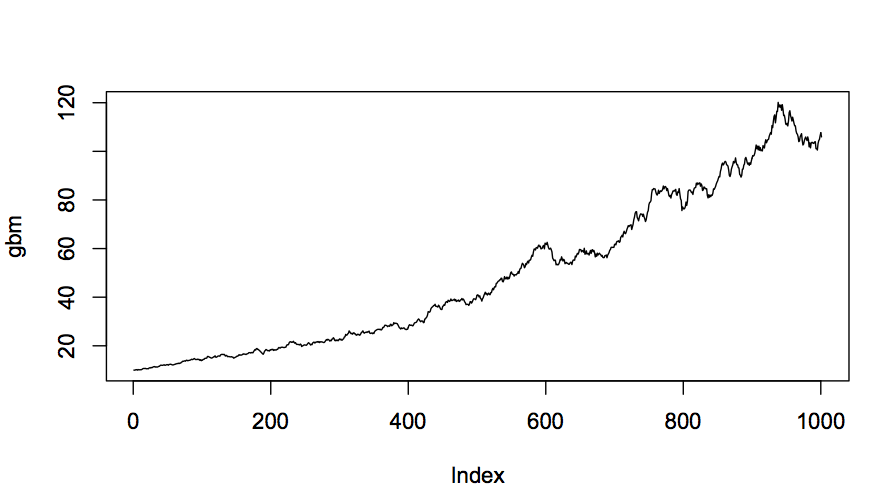Nano Particle Matrix

# npm

## stochastic

0.0.14 • Public • Published

# stochastic## Install

`npm install stochastic --save`

## Extended Usage Notes

### stoch.norm(mu, sigma, num)

Returns an array with num i.i.d normal random variables (http://en.wikipedia.org/wiki/Normal_distribution) of mean mu and standard deviation sigma.

Parameters: mu is a real number, sigma is a strictly positive real number, and num is a positive integer (defaults to 1).

### stoch.brown(mu, sigma, T, steps, path)

Returns an array corresponding to the path of Brownian motion (http://en.wikipedia.org/wiki/Wiener_process#Related_processes) from time 0 to T with drift parameter mu and volatility parameter sigma (the process is initialized to be 0). The i-th entry in the array corresponds to the Brownian process at time i * (T/steps).

Parameters: mu is a real number, sigma is a strictly positive real number, T is a strictly positive real number, steps is a positive integer, and path is a boolean. If path is false, returns only the value of the Brownian process at time T (defaults to true).

### stoch.GBM(S0, mu, sigma, T, steps, path)

Returns an array corresponding to the path of geometric Brownian motion (http://en.wikipedia.org/wiki/Geometric_Brownian_motion) from time 0 to T with drift parameter mu and volatility parameter sigma (the process is initialized to be S0). The i-th entry in the array corresponds to the geometric Brownian process at time i * (T/steps).

Parameters: S0 is a strictly positive real number, mu is a real number, sigma is a strictly positive real number, T is a strictly positive real number, steps is a positive integer, and path is a boolean. If path is false, returns only the value of the geometric Brownian process at time T (defaults to true).

### stoch.DTMC(transMatrix, steps, start, path)

Returns an array with the states at each step of the discrete-time Markov Chain (http://en.wikipedia.org/wiki/Markov_chain) given by transMatrix (2-d array). The number of transitions is given by steps. The initial state is given by start (the states are indexed from 0 to n-1 where n is the number of arrays in transMatrix).

Parameters: transMatrix is a symmetric 2-d array, steps is a positive integer, start is a non-negative integer, and path is a boolean. If path is false, returns only the value of the final state (defaults to true).

### stoch.CTMC(transMatrix, T, start, path)

Returns an object with the {key:value} pair {time:state} at each step of the continuous-time Markov Chain (http://en.wikipedia.org/wiki/Continuous-time_Markov_chain) given by transMatrix (2-d array). The Markov Chain is simulated until time T. The initial state is given by start (the states are indexed from 0 to n-1 where n is the number of arrays in transMatrix).

Parameters: transMatrix is a symmetric 2-d array, T is a strictly positive real number, start is a non-negative integer, and path is a boolean. If path is false, returns only the value of the final state (defaults to true).

### stoch.poissP(lambda, T, path)

Returns an array with the times of each arrival in a Poisson Process (http://en.wikipedia.org/wiki/Poisson_process) with rate lambda until time T.

Parameters: lambda is a strictly positive real number, T is a strictly positive real number, and path is a boolean. If path is false, returns only the number of arrivals (defaults to true).

### stoch.sample(arr, n)

Generates a random sample (with replacement) from a user input array of observations. Number of observations is specified by the user.

Parameters: arr is an array of values and n is a positive integer designating the number of observations.

### stoch.hist(arr)

Generates a histogram object from an array of data. Keys denote the lower bound of each bin and the values indicate the frequency of data in each bin.

Parameters: arr is an array of numeric values.

### stoch.exp(lambda)

Generates an exponential random variable with rate parameter lambda.

Parameters: lambda is a positive real number.

### stoch.pareto(x_m,alpha)

Generates a pareto random variables with paramters x_m and alpha.

Parameters: x_m is a positive real number and alpha is a real number.

Permission is hereby granted, free of charge, to any person obtaining a copy of this software and associated documentation files (the "Software"), to deal in the Software without restriction, including without limitation the rights to use, copy, modify, merge, publish, distribute, sublicense, and/or sell copies of the Software, and to permit persons to whom the Software is furnished to do so, subject to the following conditions:

The above copyright notice and this permission notice shall be included in all copies or substantial portions of the Software.

THE SOFTWARE IS PROVIDED "AS IS", WITHOUT WARRANTY OF ANY KIND, EXPRESS OR IMPLIED, INCLUDING BUT NOT LIMITED TO THE WARRANTIES OF MERCHANTABILITY, FITNESS FOR A PARTICULAR PURPOSE AND NONINFRINGEMENT. IN NO EVENT SHALL THE AUTHORS OR COPYRIGHT HOLDERS BE LIABLE FOR ANY CLAIM, DAMAGES OR OTHER LIABILITY, WHETHER IN AN ACTION OF CONTRACT, TORT OR OTHERWISE, ARISING FROM, OUT OF OR IN CONNECTION WITH THE SOFTWARE OR THE USE OR OTHER DEALINGS IN THE SOFTWARE.

## Keywords

### Install

`npm i stochastic`

### Repository

github.com/NathanEpstein/stochastic

### Homepage

github.com/NathanEpstein/stochastic